Next: 4.4 Discussion Up: 4. Spectral Properties of Previous: 4.2.3 Method

# 4.3 Results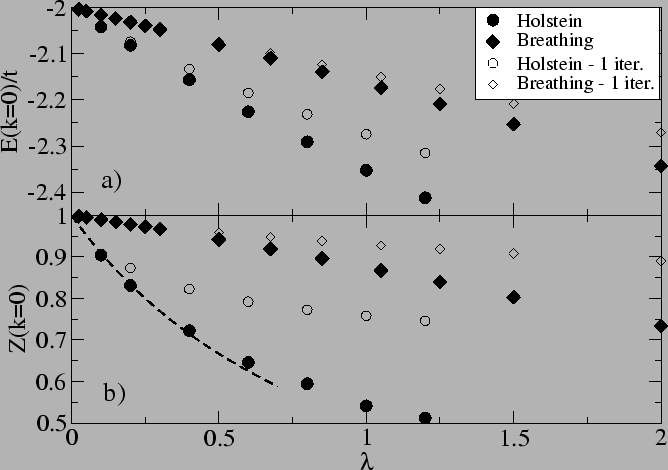In our calculations we take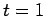and. For the one-dimensional case the coordination number.

In Fig. 4.4 -a and -b we show the polaron energyand respectively the quasiparticle weight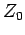versus the dimensionless coupling constantat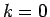. For the H model, the decrease ofwith increasingis not very different from the one given by the ME theory (dashed line in Fig. 4.4-b given by Eq. 4.16), showing that the ME definition ofis similar to ours. However, for the B model the quasiparticle weightand the polaron energydecrease much slower with increasing. As mentioned in the previous section this is due to the small momentum scattering processes implied in the renormalization of(see Eq. 4.17) and, similarly, in the determination of the self-energy. It is worth pointing out that, unlike the H polaron, even for values of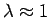, the B polaron remains in the weak coupling regime and hence the difference between the fully convergent SCBA (full symbols) and the first order perturbation theory (i.e. only the first SCBA iteration, empty symbols) is small. Another interesting feature is that for the same value ofthe B polaron energy is lower than the H one, showing that the ratio between the energy renormalization and the quasiparticle weight renormalization is different for the two models.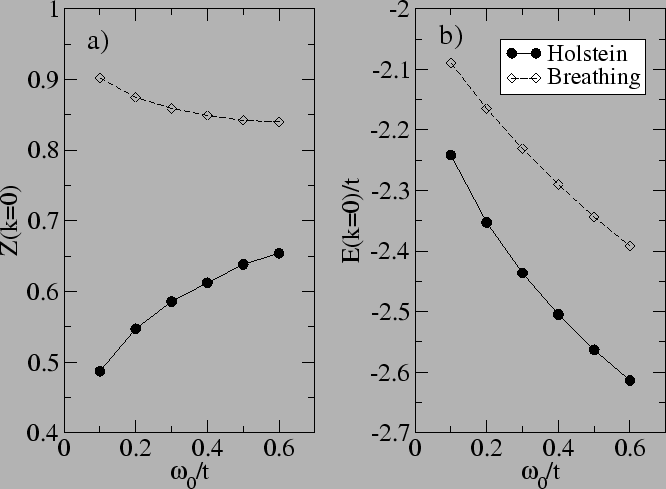Another important difference between the two models is the dependence of the polaron properties at the band bottom on the phonon frequency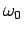. While for the H case an increase ofresults in an increase of the quasiparticle weightthe opposite behavior is seen for the B model. This is illustrated in Fig. 4.5-a. The reason for the reduction ofwith increasingin the B model can be easily understood by noticing (see Eq. 4.17) that a larger value ofreduces the importance of thedependence in the polaron properties calculation. As discussed earlier, the strong momentum dependent coupling is responsible for the weakrenormalization of the B polaron and thus an increase ofwould result in a larger effective coupling and implicitly in a smaller.Aside from the differentanddependency of the two models at zero momentum, the momentum dependent properties also exhibit different behaviors. This is shown in Figs. 4.64.74.8 and  4.9 where thedependent properties for the two models are illustrated.

In Fig. 4.6 and Fig. 4.7-a and -b we have chosen the value ofsuch that both models yield the same quasiparticle weight at the bottom of the band. Thus, the choice of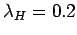and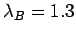results in, implying that both models are in the weak coupling regime. As mentioned earlier, the B polaron energy atis lower. At large, just below the first phonon threshold energy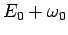, both polarons display a flat dispersion and a reduced quasiparticle weight. However, the B polaron quasiparticle weight at largeis substantially larger (for instance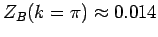within a numerical precision of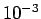and), making the B polaron state at largedistinguishable in the spectral plot (Fig. 4.7 -b) in contrast to the H one (Fig. 4.7-a). At energies larger thanthe spectral intensity of the B quasiparticle is much smaller than the H model one, unlike the situation at the band bottom where both models have the same. This large momentum behavior points to a stronger effective coupling for the B model at large.

While the dispersion of both models displays a gap at, the B polaron shows a second gap at the second phonons threshold energy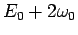. This can be seen in Fig. 4.7-b but also occurs for smaller values of the dimensionless coupling as shown in Fig. 4.7-c for the value of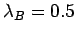. This value was chosen such that the ground state energyof the B model is equal to that of the Holstein polaron one shown in Fig. 4.7-a. The situation can be even clearer visualized by comparing Fig. 4.8-a with Fig. 4.8-b, where the energy distribution curves (EDC) for H and respectively B cases are shown.

An even more interesting effect is noticed if a linear dispersion for the free electron is considered(4.24)

with a value of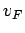close to one or larger. In this case one can taketo be a measure of the free electron kinetic energy 4.2 and thus define the dimensionless coupling as(4.25)

The resulting B polaron dispersion is shown in Fig. 4.7-d (see also the corresponding EDC plot in Fig. 4.9-b). While it displays a gap at, no distinguishable gap or kink can be seen at the first phonon threshold energy. This free-electron like behavior of the polaron atis due to the fact that the physics there is determined by very smallscatterings, characterized by small coupling strength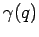, originating from the rapid increase of the electron energy with. At larger energy, close to, the relevant phonon momentaimplied in the scattering are larger and the physics is consequently determined by a larger effective coupling. As a result a noticeable kink appears at this energy in the spectrum. This effect (i.e. kink atbut no noticeable one at) is a result of an electron-phonon coupling which is an increasing function of the polaron momentum and it is hence not seen in the Holstein model even for the case of a linear electronic dispersion (see Fig. 4.7-e and the corresponding EDC plot in Fig. 4.9-a).

The differences between the two models discussed above are a consequence of two effects: i) the strongdependence of the bare electron-phonon couplingin the B model and ii) the polaron properties at smallare most strongly influenced by the small momentumphonons. However the second statement is not true if the free electron dispersion has low energy states separated by largeas we will discuss in the next section (Sec.4.4). In order to show this we choose a free electron dispersion(4.26)

which is double degenerate with the lowest energy values atand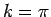respectively. We find that for this electronic dispersion the differences between the H and the B model are very small, less than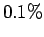, and therfore not discernible in the spectral representation plot shown in Fig. 4.7-f.

The general features of the polaron spectral function illustrated in Figs. 4.8 and 4.9 show a remarkable resemblance with the photoemission data in materials with significant electron-phonon interactions (2,19). For momenta which correspond to energies below the phonon frequency one can see a sharp peak followed by a broad satellite. At large momenta, the broad satellite found at energies well above the phonon frequency sharpens and follows the free electron dispersion while the intensity of the sharp peak belowvanishes. We want to point that this picture, with the spectral function determined by two main branches, is quite different form the one incorrectly presented in some popular textbooks (38) where the spectrum is characterized by a single quasiparticle peak which changes its dispersion slope when crossing the phonon energy threshold.Next: 4.4 Discussion Up: 4. Spectral Properties of Previous: 4.2.3 Method
© Cyrill Slezak